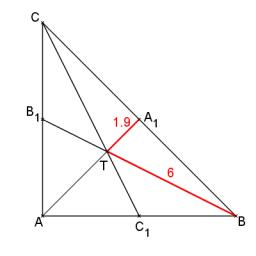# Calculate 4424

Calculate the area of the triangle ABC if a = 10 cm, c = 8 cm, ta = 6 cm.

S =  29.9625 cm2

### Step-by-step explanation:

Try calculation via our triangle calculator.Did you find an error or inaccuracy? Feel free to write us. Thank you!

Tips for related online calculators